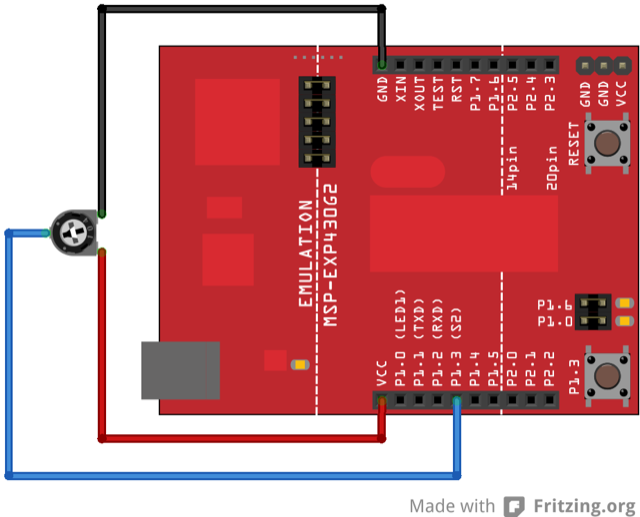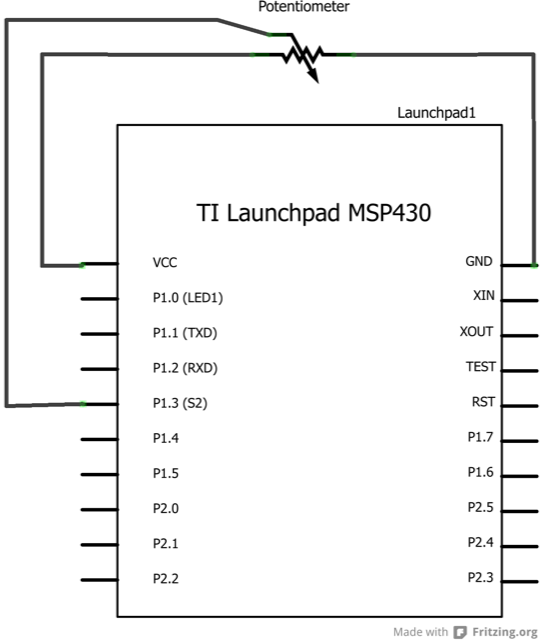## Analog Read Voltage

This example shows you how to read an analog input on Pin A3, convert the values from `analogRead()` into voltage, and print it out to the serial monitor.

### Hardware Required

• A variable resistor, like a potentiometer

### Relevant Groundwork

Connect the three wires from the potentiometer to your LaunchPad board. The first goes to ground from one of the outer pins of the potentiometer. The second goes from 3 volts to the other outer pin of the potentiometer. The third goes from analog input A3 to the middle pin of the potentiometer.

By turning the shaft of the potentiometer, you change the amount of resistance on either side of the wiper which is connected to the center pin of the potentiometer. This changes the voltage at the center pin. When the resistance between the center and the side connected to 3 volts is close to zero (and the resistance on the other side is close to 10 kilohms), the voltage at the center pin nears 3 volts. When the resistances are reversed, the voltage at the center pin nears 0 volts, or ground. This voltage is the analog voltage that you’re reading as an input.

The LaunchPad has a circuit inside called an analog-to-digital converter that reads this changing voltage and converts it to a number between 0 and 1023. When the shaft is turned all the way in one direction, there are 0 volts going to the pin, and the input value is 0. When the shaft is turned all the way in the opposite direction, there are 3 volts going to the pin and the input value is 1023. In between, analogRead() returns a number between 0 and 1023 that is proportional to the amount of voltage being applied to the pin.

### CircuitImage developed using Fritzing. For more circuit examples, see the Fritzing project page.

### Schematic### Code Explanation

In the program below, the very first thing that you do will in the setup function is to begin serial communications, at 9600 bits of data per second, between your LaunchPad and your computer with the line:

`Serial.begin(9600);`

Next, in the main loop of your code, you need to establish a variable to store the resistance value (which will be between 0 and 1023, perfect for an int datatype) coming in from your potentiometer:

`int sensorValue = analogRead(A3);`

To change the values from 0-1023 to a range that corresponds to the voltage the pin is reading, you’ll need to create another variable, a int, and do a little math. `int` is recommended over float on MSP430 (and microcontrollers in general) because it uses less memory. Memory constraints are a big concern when programming microcontrollers. It is very easy to run out of it, so always be careful what datatypes and variables are declared in the program. To scale the numbers between 0.0 and 3.0, divide 3.0 by 1023.0 and multiply that by sensorValue:

`int voltage= sensorValue * (3.0 / 1024.0);`

Finally, you need to print this information to your serial window as. You can do this with the command Serial.println() in your last line of code:

`Serial.println(voltage);`

Now, when you open your Serial Monitor in the Energia development environment (by clicking the button directly to the right of the ''Upload'' button in the header of the program), you should see a steady stream of numbers ranging from 0.0-3.0. As you turn the pot, the values will change, corresponding to the voltage coming into pin A3.

### Code

```/*
Reads an analog input on pin A3, converts it to voltage, and prints the result to the serial monitor.
Attach the center pin of a potentiometer to pin A3, and the outside pins to +3V and ground.

Hardware Required:
* Potentiometer

This example code is in the public domain.
*/

// the setup routine runs once when you press reset:
void setup() {
// initialize serial communication at 9600 bits per second:
Serial.begin(9600); // msp430g2231 must use 4800
}

// the loop routine runs over and over again forever:
void loop() {
// read the input on analog pin A3:
int sensorValue = analogRead(A3);
// Convert the analog reading (which goes from 0 - 1023) to a voltage (0 - 3V):
// Can either use type int or float to store voltage, int takes up less memory and is recommend
// Memory is a huge concern when programming microcontollers, be careful what datatypes are used
// in order to make the most of the available memory
int voltage = sensorValue * (3.0 / 1023.0);
// You can compare the size of the code by running the program using int and then running with float
// You will see ~4k bytes for int vs ~6k bytes for float just by changing the datatype, quite astonishing.
//float voltage = sensorValue * (3.0 / 1023.0);

// print out the value you read:
Serial.println(voltage);
}```

### Try it out

• Use a potentiometer to manipulate an LED.